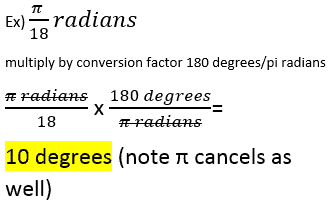Most students in Algebra II are comfortable using degrees, as that was typically included in their previous geometry course. Radians are an alternative way to measure angles and their refer to the length of the arc on the unit circle for a given angle.

For example, a 45 degree angle corresponds to π/4 radians.  Think of it like this: the circumference of a circle is 2πr and with a unit circle having a radius of 1, the circumference is 2π. Thus, a circle has 360 degrees or 2πr. Now, an 1/8 of a circle gives you a 45 degree angle or π/4 (2π/8).

To convert from degrees to radians, multiply degrees by π/180. And to convert from radians to degrees, multiply radians by 180/π.Another way to think convert involves conversion factors. Cancel units that you begin with to get to the unit you’d like (either degrees or radians). Here’s an example: